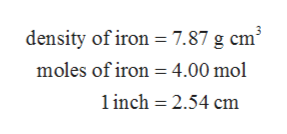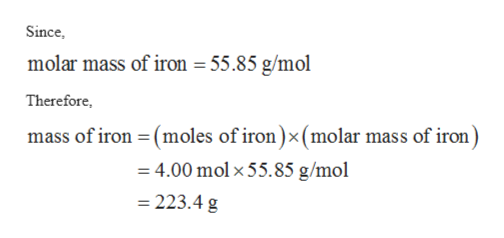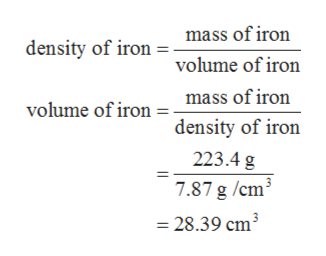# Iron has a density of 7.87 g cm3 . What is the volume (in cubic inches) of an iron sample which contains 4.00 moles of iron? 2.54 cm = 1 inch (exact conversion)

Question

Iron has a density of 7.87 g cm3 . What is the volume (in cubic inches) of an iron sample which contains 4.00 moles of iron? 2.54 cm = 1 inch (exact conversion)

check_circleExpert Solution
Step 1

The values are given as follows:help_outlineImage Transcriptionclosedensity of iron 7.87 g cm3 moles of iron 4.00 mol inch 2.54 cm fullscreen
Step 2

The mass of iron can be calculated with the help of molar mass of iron as follows:help_outlineImage TranscriptioncloseSince molar mass of iron 55.85 g/mol Therefore mass of iron (moles of iron)x (molar mass of iron) 4.00 mol x 55.85 g/mol 223.4 g fullscreen
Step 3

The volume of iron can be calculated with th...help_outlineImage Transcriptionclosemass of iron density of iron = volume of iron mass of iron volume of iron density of iron 223.4 g 7.87 g /cm =28.39 cm3 fullscreen

### Want to see the full answer?

See Solution

#### Want to see this answer and more?

Solutions are written by subject experts who are available 24/7. Questions are typically answered within 1 hour*

See Solution
*Response times may vary by subject and question
Tagged in

### General Chemistry